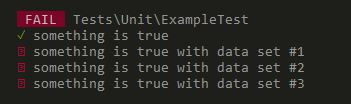#DAY 3
0
Modern Web

## 03. Unit Test x PHPUnit x FizzBuzz

Fizz Buzz 是個小朋友的遊戲，小朋友們依序報數，但當遇到三的倍數要喊 fizz、五的倍數喊 fuzz，遇到既是三也是五的倍數要喊 fizzbuzz。

### 來玩 FizzBuzz ㄅ

Unit Test

tests/Unit 裡面有一個內建的 ExampleTest 檔案。
assertTrue() 會檢查傳入的參數是否是 true，是則回傳 true。

``````<?php

namespace Tests\\Unit;

use PHPUnit\\Framework\\TestCase;
use App\\My\\Service\\TestService;

class ExampleTest extends TestCase
{
public function testSomethingIsTrue()
{
\$this->assertTrue(true);
}
}

``````

``````public function testSomethingIsTrue()
{
\$input = "1";
\$output = \$this->fizzbuzz(\$input);
\$this->assertSame(\$input,\$output);
}

private function fizzbuzz(\$input) {
return \$input;
}

``````

`````` /**
* @dataProvider data
*/
public function testSomethingIsTrue(\$input, \$expect)
{
\$output = \$this->fizzbuzz(\$input);
\$this->assertSame(\$output, \$expect);
}

public function data()
{
return [
[ "1", "1" ],
[ "3", "fizz" ],
[ "5", "buzz" ],
[ "15", "fizzbuzz" ]
];
}

private function fizzbuzz(\$input) {
return \$input;
}

````````````private function fizzbuzz(\$input) {

\$input = (int) \$input;

if( \$input % 15 == 0 ){
return 'fizzbuzz';
}

if( \$input % 3 == 0 ){
return 'fizz';
}

if( \$input % 5 == 0 ){
return 'buzz';
}

return (string) \$input;

}

``````

### AAA pattern

Arrange = 準備受測物件、參數、預期結果
Act = 執行受測方法
Assert = 驗證執行結果與預測結果是否一致

0

fizz
buzz
fizzbuzz~~~~